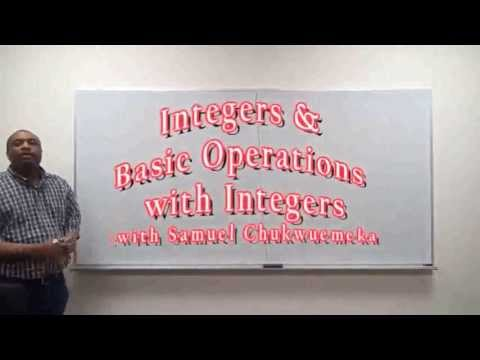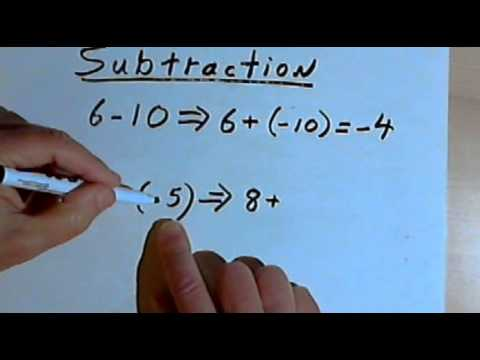## Number System

Subject: Optional Maths

#### Overview

Mathematics is the study of numbers which is start from the number system . This note gives information about the number system and surds.And even Natural numbers, Integers , Rational number , irrational numbers etc..

### Number System

Mathematics is the study of numbers and it starts from the number system. According to our level, we shall start from Real numbers. The chart below explains about the number system:

#### Natural numbers ( N )

You can see a number line having a set of numbers are positive. In the figure the possesses will never end if we continue the process.So, marked numbers are called natural numbers which are denoted by N.Here, the natural numbers are 1, 2, 3, 4, 5......

1. The first natural numbers is 1 which is also the least natural number.
2. The next natural numbers can be obtained adding 1 to any natural number.

#### Zero

Before only numbers except zero has been used. Zero usually means the emptiness which is denoted by 0. It is middle numbers between positive and negative numbers.

#### Whole numbers ( W )

A set of the natural number along with zero is called whole numbers which are denoted by W. Hence, whole numbers W = 0, 1, 2, 3, . . . . . .1. It is the first and the least whole number is 0.
2. It is next whole number which is obtained by adding 1 to any whole number

#### Integers ( $\mathbb{Z}$)

We know ,
Natural numbers are = 1, 2, 3, 4, 5, ....... and Negative natural numbers are = -1, -2, -3, -4, -5, ......

Therefore, the set of all positive natural numbers, negative natural numbers inclusive of zero is known as integers. It is denoted by $\mathbb{Z}$.

So, $\mathbb{Z}$ = ........, - 3, - 2, - 1, 0, 1, 2, 3, .......

figure

Note :

1. Positive integers : ( $\mathbb{Z}$+ )
The set of all natural numbers is known as positive integers.It is denoted by $\mathbb{Z}$+ .
∴ Z= 1, 2, 3, 4, ............ = N.
2. Negative Integers: ( $\mathbb{Z}$):
The set of all negative natural numbers is known as integers.It is denoted by $\mathbb{Z}$
∴ Z= - 1, - 2, - 3, .........
3. O ( Zero ) is neither positive integers nor negative integer .It is a natural number.

#### Rational Number (Q)

A number that can be written in the form of $\frac{p}{q}$ where q ≠ o is called rational number .It is derived from the word ratio which also satisfies the structure $\frac{p}{q}\}. Note:p and q also needs to be integers eg: \(\frac{2}{3}$ , $\frac{5}{9}$, 0 etc .

Properties of rational numbers

1. Here, decimals of rational members are either terminating or recurring .Any rational number can be written in a ratio .Hence, it was divided .When they are divided the decimals are either terminating or repeating.
Eg: $\frac{1}{2}$
$\require{enclose}\begin{array}{r}0.5\\[-3pt]2\enclose{longdiv} {10}\\[-3pt]\underline{−10} \end{array}$
Divident = 1
Divisor = 2
Here, $\frac{1}{2}$ = 0.5 which is terminating decimal.

#### Irrational Numbers ($\overline{Q}$ )

Those members which can not be expressed in the form of $\frac{a}{b} \} where a and b both are integers and b ≠ 0 , are called irrational numbers . eg : \(\sqrt{5}$, $\sqrt{3}$, 5$\sqrt{7}$, $\sqrt{3}$+2, $\frac{1}{2 + \sqrt{5}}$,π, 0.303003000 . . . . .
The decimals of irrational numbers are neither terminating nor repeating.

• Nomenclature of an Irrational Number

eg: In $\sqrt{2}$ the order of $\sqrt{2}$ is 2.
In $\sqrt{29}$ the order of $\sqrt{29}$ is 3.

##### Things to remember
• Positive and negative integers are denoted by ( Z+ ) and ( Z- )  respectively.
• 0 ( Zero ) is neither positive integers nor negative integers .It is  a natural number.
• Here, decimals of rational  members  either terminating or recurring .
• It includes every relationship which established among the people.
• There can be more than one community in a society. Community smaller than society.
• It is a network of social relationships which cannot see or touched.
• common interests and common objectives are not necessary for society.
##### Videos for Number System##### Add, Subtract, Multiply and Divide Integers##### Rules for Positive and Negative Numbers

Solution

$\frac{2}{5}$

= $\require{enclose}\begin{array}{r}0.4\\[-3pt]5\enclose{longdiv} {20}\\[-3pt]\underline{20} \end{array}$

Here, Divident = 2

Divisor = 5

Solution

$\frac{1}{2}$

= $\require{enclose}\begin{array}{r}0.5\\[-3pt]2\enclose{longdiv} {10}\\[-3pt]\underline{10} \end{array}$

Divident = 1

Divisor = 2

Here, $\frac{1}{2}$= 0.5 which is terminating decimal.

Solution

= $\frac{11}{30}$

= $\require{enclose}\begin{array}{r}0.366\\[-3pt]30\enclose{longdiv}{110}\\[-3pt]\underline{90}\\[-3pt]{200} \\[-3pt]\underline{180}\\[-3pt]\\{200}\\[-3pt]\underline{180}\\[-3pt]{20}\end{array}$

Divident= 11

Divisor= 30

Here, $\frac{11}{30}$ = 0.366 is also repeating decimal. So it is rational number.

Solution

=$\frac{7}{22}$

= $\require{enclose}\begin{array}{r}1.31818\\[-3pt]22\enclose{longdiv}{70}\\[-3pt]\underline{66}\\[-3pt]{40} \\[-3pt]\underline{22}\\[-3pt]\\{180}\\[-3pt]\underline{176}\\[-3pt]{40}\\[-3pt]\underline{22}\\[-3pt]{180}\\[-3pt]\underline{176}\\{4}\end{array}$

Divident= 7

Divisor= 22

Here, $\frac{7}{22}$ = 0.31818 which is repeating decimal. So, $\frac{7}{22}$ is a rational number.

The set of all the natural along with zero is known as hole numbers.

Any number that can be written in the form of $\frac{p}{q}) where q≠0 is known as the rational number. Zero(0) is neither positive integers nor negative integer. It is a natural number. The positive integers are those whose set are all positive natural numbers and it is denoted by Z+. Integers are the set of all positive and negative natural numbers inclusive of Zero. I t can be denoted by Zero(0). Solution =\(\frac{1}{4}$

=$\require{enclose}\begin{array}{r}0.25\\[-3pt]4\enclose{longdiv}{10}\\[-3pt]\underline{8}\\[-3pt]{20}\\\underline{20}\end{array}$

Divident = 1

Divisor= 4

Here, $\frac{1}{4}$ = 0.25 which is terminating decimal.

Solution

$\frac{1}{4}$

= $\require{enclose}\begin{array}{r}0.25\\[-3pt]4\enclose{longdiv} {10}\\[-3pt]\underline{8} \\[-3pt]20\\\underline{20}\end{array}$

=  Here, the quotient is 0.25. It is terminating after 2 decimals.

Divident= 1

Divisor = 4

Solution

=$\frac{2}{9}$

= $\require{enclose}\begin{array}{r}0.222\\[-3pt]9\enclose{longdiv}{20}\\[-3pt]\underline{18}\\[-3pt]{20} \\[-3pt]\underline{18}\\[-3pt]\\{20}\\[-3pt]\underline{18}\end{array}$

Divident = 2

Divisor = 9

Here, $\frac{2}{9}$ = 0.222 which is repeating decimal. So, it is also a rational number.

Solution

= $\frac{13}{8}$

= $\require{enclose}\begin{array}{r}1. 625\\[-3pt]8\enclose{longdiv}{13}\\[-3pt]\underline{8}\\[-3pt]{50} \\[-3pt]\underline{48}\\[-3pt]\\{20}\\[-3pt]\underline{16}\\[-3pt]{40}\\[-3pt]\underline{40}\\{0}\end{array}$

Divident = 13

Divisor = 8

Here, $\frac{13}{8}$ = 1.625 which is terminating decimal.

The properties of rational numbers are listed below:

• Any rational numbers can be written in a ratio.
• It must be divided.
• The decimals of rational members are either terminating or recurring.

Solution

=$\frac{1}{8}$

= $\require{enclose}\begin{array}{r}0.25\\[-3pt]8\enclose{longdiv}{10}\\[-3pt]\underline{8}\\[-3pt]{20}\\[-3pt]\underline{16}\\[-3pt]{40}\\\underline{40}\end{array}$

Here, Divident= 1

Divisor = 8

Since, $\frac{1}{8}$ = 0.25 which is terminating decimal. So, it is rational number.